# Geometric Series Example

Previous: The Geometric Series

Next: Converting an Infinite Decimal Expansion to a Rational Number

## Example

Determine if the following series is convergent, and if so, find its sum:## Complete Solution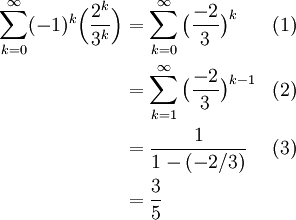The sum of the series is therefore 3/5.

## Explanation of Each Step

### Step (1)

We first rewrite the problem so that the summation starts at one and is in the familiar form of a geometric series, whose general form isAfter bringing the negative one and the three fifths together, we see that our given infinite series is geometric with common ratio -3/5.

For a geometric series to be convergent, its common ratio must be between -1 and +1, which it is, and so our infinite series is convergent.

We must now compute its sum.

### Step (2)

The given series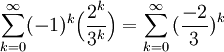starts the summation at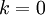, so we shift the index of summation by one: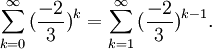Our sum is now in the form of a geometric series with a = 1, r = -2/3. Since |r| < 1, the series converges, and its sum is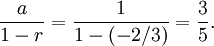### Step (3)

In Step (3) we applied the formula for the sum of a geometric series: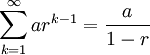This formula was derived in a previous section of this lesson.

## Possible Challenge Areas

### Step (2)

Students who may have been confused by this step may wish to refer to the previous lesson on Sigma Notation, where this process was explained in more detail.

Previous: The Geometric Series

Next: Converting an Infinite Decimal Expansion to a Rational Number